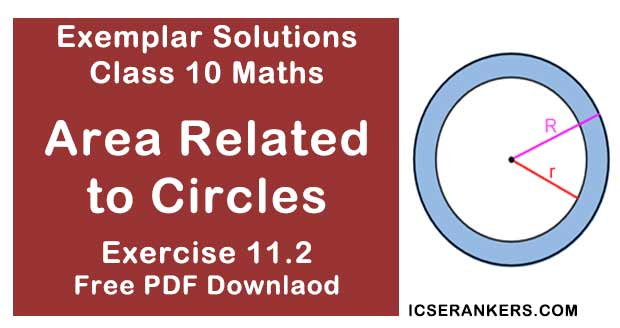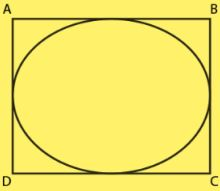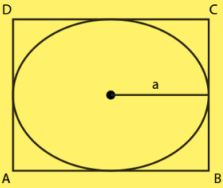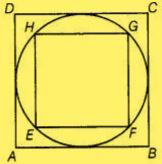# Chapter 11 Area Related to Circles NCERT Exemplar Solutions Exercise 11.2 Class 10 MathsChapter Name NCERT Maths Exemplar Solutions for Chapter 11 Area Related to Circles Exercise 11.2 Book Name NCERT Exemplar for Class 10 Maths Other Exercises Exercise 11.1Exercise 11.3Exercise 11.4 Related Study NCERT Solutions for Class 10 Maths

### Exercise 11.2 Solutions

1. Is the area of the circle inscribed in a square of side a cm, πa2 cm2 ? Give reasons for your answer.

Solution

False
Justification:
Let a be the side of square.
We have,
The circle is inscribed in the square.Diameter of circle  = Side of square = a
Radius of the circle = a/2
Area of the circle = πr2
= π(a/2)2
= πa2/4 cm2
Therefore, area of the circle is πa2/4 cm2
So, the area of the circle inscribed in a square of side a cm is not a2 cm2

2. Will it be true to say that the perimeter of a square circumscribing a circle of radius a cm is 8a cm? Give reasons for your answer.

Solution

True
Justification:
Let r be the radius of circle = a cmDiameter of the circle = d = 2 × Radius = 2a cm
As the circle is inscribed in the square,
Side of a square = Diameter of circle = 2a cm
Therefore, Perimeter of a square = 4 × (side)
= 4 × 2a
= 8a cm
Hence, it will be true to say that the perimeter of a square circumscribing a circle of radius a cm is 8a cm.

3. In Fig., a square is inscribed in a circle of diameter d and another square is circumscribing the circle. Is the area of the outer square four times the area of the inner square? Give reasons for your answer.Solution
False
Justification:
Diameter of the circle = d
So,
Diagonal of inner square EFGH = Side of the outer square ABCD
= Diameter of circle
= d
Let us take the side of inner square EFGH be 'a'.
Now in right angled triangle EFG,
(EG)2 = (EF)2 + (FG)2
[By Pythagoras theorem]
d2 = a2 + a2
⇒ d2 = 2a2
⇒ a2 = d2/2
Area  of inner circle = a2
= d2/2
Also,
Area of outer square = d2
Therefore, the area of the outer circle is only two times the area of the inner circle.
Thus, area of outer square is not equal to four times the area of the inner square.

4. Is it true to say that area of a segment of a circle is less than the area of its corresponding sector? Why?
Solution
False
Justification :
It is not true because in case of major segment, area is always greater than the area of its corresponding sector. It is true only in the case of minor segment.
Therefore, we can conclude that it is not true to say that area of a segment of a circle is less than the area of its corresponding sector.

5. Is it true that the distance travelled by a circular wheel of diameter d cm in one revolution is
2πd cm? Why?
Solution
False
Justification :
Distance travelled by a circular wheel of radius r in one revolution equals the circumference
of the circle.
We have,
Circumference of the circle = 2πd [where d is the diameter of the circle]
Hence, it is not true that the distance travelled by a circular wheel of diameter d cm in one revolution is 2d cm.

6. In covering a distance s metres, a circular wheel of radius r metres makes s/2πr revolutions. Is this statement true ? Why?
Solution
True
Justification:
The distance travelled by a circular wheel of radius r m in one revolution is equal to the
circumference of the circle = 2πr
No. of revolutions completed in 2πr m distance = 1
No. of revolutions completed in 1 m distance = 1/2πr
No. of revolutions completed in s m distance = (1/2πr) × s
= s/(2πr)
Hence, the statement "in covering a distance s metres, a circular wheel of radius r metres  makes s/2πr revolutions" is correct.

7. The numerical value of the area of a circle is greater than the numerical value of its circumference. Is this statement true? Why?
Solution
False
Justification,
If 0 < r < 2, then numerical value of circumference is greater than numerical value of area of circle and if r > 2, area is greater than circumference.

8. If the length of an arc of a circle of radius r is equal to that of an arc of a circle of radius 2 r, then the angle of the corresponding sector of the first circle is double the angle of the corresponding sector of the other circle. Is this statement false? Why?
Solution
False
Justification,
Let two circles C1 and C2 of radius r and 2r with centres O and O‟, respectively.
Given,
Length of an arc of a circle of radius r = Length of an arc of a circle of radius 2 r
Or,
Length AB = Length CD
Let,
Angle subtended by arc AB = θ1
Therefore,
AB =  (θ1/360) × 2πr
Also,
Angle subtended by arc CD = θ2
Therefore,
CD =  (θ2/360) × 2π(2r)
⇒ CD =  (θ2/360) × 4πr
We above equations,
1/360) × 2πr = (θ2/360) × 4πr
⇒ θ1 = 2θ2
Therefore, angle of the corresponding sector of C1 is double the angle of the corresponding sector of C2.
It is true statement

9. The areas of two sectors of two different circles with equal corresponding arc lengths are equal. Is this statement true? Why?
Solution
False
Justification,
It is true for arcs of the same circle. But in different circle, it is not possible.

10. The areas of two sectors of two different circles are equal. Is it necessary that their corresponding arc lengths are equal? Why?
Solution
False
Justification,
It is true for arcs of the same circle. But in different circle, it is not possible.

11. Is the area of the largest circle that can be drawn inside a rectangle of length a cm and breadth b cm (a > b) is πb2 cm2? Why?
Solution
False
Justification,
The area of the largest circle that can be drawn inside a rectangle is π(b/2)2 cm, where π(b/2) is the radius of the circle and it is possible when rectangle becomes a square.

12. Circumferences of two circles are equal. Is it necessary that their areas be equal ? Why ?
Solution
True
Justification,
If circumference of two circles are equal, then their corresponding radii are equal. So, their areas will be equal.

13. Areas of two circles are equal. Is it necessary that their circumferences are equal? Why?
Solution
True
Justification :
If areas of two circles are equal, then their corresponding radii are equal. So, their circumference will be equal.

14. Is it true to say that area of a square inscribed in a circle of diameter p cm is p2 cm2? Why?
Solution
False
Justification,
When the square is inscribed in the circle, the diameter of a circle is equal to the diagonal of a
square but not the side of the square.
So, we cannot say that area of a square inscribed in a circle of diameter p cm is p2 cm2.